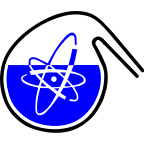#RadChem 2018

13-18 May 2018
Casino Conference Centre
Europe/Prague timezone

## Crystal structure and solubility of radium sulfate and carbonate

18 May 2018, 11:45
15m
Red Hall (Casino Conference Centre)

### Red Hall

#### Casino Conference Centre

Reitenbergerova 4/95, Mariánské Lázně, Czech Republic
Verbal Chemistry of Nuclear Fuel Cycle, Radiochemical Problems in Nuclear Waste Management

### Speaker

Artem Matyskin (Chalmers University of Technology)

### Description

Radium is the heaviest alkaline-earth metal. It has no stable isotopes and the most long-lived and abundant isotope is $^{226}$Ra with a half-life of 1600 years. Radium and its short-lived daughters are among the most radiotoxic elements. Enhanced radium concentrations can be found in different waste streams: uranium and other metal mining, fossil fuel combustion, oil and gas production among others. Moreover, radium ingrowths occur in spent nuclear fuel, which makes it a critical element for long-term safety assessment of final nuclear waste repositories . One of the main factors controlling radium migration in the environment is its co-precipitation with other alkaline-metal sulfate phases, for example barite (BaSO$_{4}$). In some natural sulfate free waters, radium mobility can be controlled by its co-precipitation with carbonate minerals, for example, witherite (BaCO$_{3}$) . The mechanism and degree of radium co-precipitation with barite and witherite depends mainly on crystal structure and the solubilities of pure barite, witherite, RaSO$_{4}$ and RaCO$_{3}$. The crystal structure and solubility of barite, witherite and other non-radioactive alkaline-earth metal sulfate and carbonate phases are well established, but little is known about the properties of RaSO$_4$ and RaCO$_3$. The objective of this work was to study the crystal structures and solubilities of radium-barium sulfate and carbonate and obtain more insight into radium co-precipitation mechanisms and the migration of radium in the environment.
For this purpose, an old radium source was disassembled to obtain radium-barium sulfate (Ra$_{0.76}$Ba$_{0.24}$SO$_{4}$). Radium-barium carbonate (Ra$_{0.76}$Ba$_{0.24}$CO$_{3}$) was synthesized from the Ra$_{0.76}$Ba$_{0.24}$SO$_{4}$ obtained by three cycles of Ra$_{0.76}$Ba$_{0.24}$SO$_{4}$ heating in 1.5 mol·L$^{-1}$ Na$_2$CO$_3$ up to 85 °C, cooling and subsequent removal of supernatant . The Ra$_{0.76}$Ba$_{0.24}$SO$_{4}$ obtained was characterized by an X-ray Diffraction (XRD) technique. Rietveld refinement showed that Ra$_{0.76}$Ba$_{0.24}$SO$_{4}$ crystallizes in the Pnma (62) space group and is isostructural with pure barite (BaSO$_4$). Density Functional Theory (DFT) and Vegard’s law were used to obtain atomic coordinates and unit cell parameters of pure RaSO$_4$ (a=9.129(8), b=5.538(3), c=7.313(5)) . The synthesized Ra$_{0.76}$Ba$_{0.24}$CO$_3$ was characterized by XRD and Extended X-Ray Absorption Fine Structure (EXAFS) techniques. EXAFS refinement indicated that radium is nine-coordinate in solid Ra$_{0.76}$Ba$_{0.24}$CO$_3$ with a mean Ra-O bond distance of 2.885(5) Å. Comparison of the Ra$_{0.76}$Ba$_{0.24}$CO$_3$ and witherite (BaCO$_3$) XRD patterns showed these solids are not isostructural.
The solubilities of Ra$_{0.76}$Ba$_{0.24}$SO$_4$ and Ra$_{0.76}$Ba$_{0.24}$CO$_3$ were measured from over- and undersaturation as a function of ionic strength (NaCl media). The concentration of radium was measured by gamma spectrometry after separation of the aqueous phase from colloids using ultracentrifugation to calculate its concentration and the apparent solubility product. The extended specific ion interaction theory (ESIT) was used to extrapolate the apparent solubility product of Ra$_{0.76}$Ba$_{0.24}$SO$_4$ and Ra$_{0.76}$Ba$_{0.24}$CO$_3$ to zero ionic strength and obtain the Ra$^{2+}$ - Cl$^{-}$ ion interaction coefficients. It was shown that the apparent solubility products of Ra$_{0.76}$Ba$_{0.24}$SO$_4$ and BaSO$_4$ as well as those of Ra$_{0.76}$Ba$_{0.24}$CO$_3$ and BaCO$_3$ increase almost identically from zero ionic strength with an increase in the ionic strength of NaCl which indicates that Ra$^{2+}$ and Ba$^{2+}$ undergo similar short-range interactions with Cl$^-$ in aqueous media as might be expected given the similar ionic radii of Ba$^{2+}$ and Ra$^{2+}$. The solubility of Ra$_{0.76}$Ba$_{0.24}$SO$_4$ at zero ionic strength is a little lower (0.2 log$_{10}$ units) than the solubility of pure barite (BaSO$_4$). The results reveal that both the solubility and crystal structure of Ra$_{0.76}$Ba$_{0.24}$SO$_4$ is very similar to the solubility and crystal structure of barite and that radium and barium form an ideal solid solution in the sulfate phase. The solubility of Ra$_{0.76}$Ba$_{0.24}$CO$_3$ at zero ionic strength is significally higher (1 log$_{10}$ unit) than the solubility of pure witherite (BaCO$_3$). This confirms that Ra$_{0.76}$Ba$_{0.24}$CO$_3$ and pure RaCO$_3$ are not isostructural with witherite (BaCO$_3$).

 Grandia, F., Merino, J., & Amphos, J. B. (2008). Assessment of the radium-barium co-precipitation and its potential influence on the solubility of Ra in the near-field. Stockholm, Sweden: SKB.
 Langmuir, D., & Riese, A. C. (1985). The thermodynamic properties of radium. Geochimica et cosmochimica acta, 49(7), 1593-1601.
 Matyskin, A. V., Ebin, B., Tyumentsev, M., Allard, S., Skarnemark, G., Ramebäck, H., & Ekberg, C. (2016). Disassembly of old radium sources and conversion of radium sulfate into radium carbonate for subsequent dissolution in acid. Journal of Radioanalytical and Nuclear Chemistry, 310(2), 589-595.
 Matyskin, A. V., Ylmen, R., Lagerkvist, P., Ramebäck, H., & Ekberg, C. (2017). Crystal structure of radium sulfate: an X-ray powder diffraction and density functional theory study. Journal of Solid State Chemistry, 253, 15-20.

### Primary authors

Artem Matyskin (Chalmers University of Technology) Dr Paul L. Brown (Rio Tinto Growth and Innovation) Christian Ekberg (Chalmers University of Technology)

### Presentation Materials

There are no materials yet.